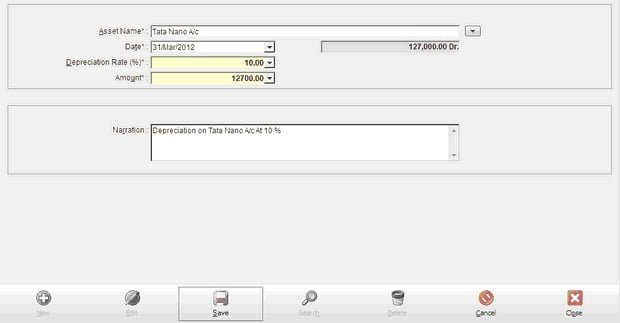You can add details of asset depreciation in this module. First select asset name from the list,enter depreciation rate in percentage now calculate depreciation amount show.Asset Name Select asset on which you have to calculate depreciation. Date Date of transaction. Depreciation Rate (%) Enter depreciation rate in percentage. Amount Calculate amount show in amount. Narration Enter narration.